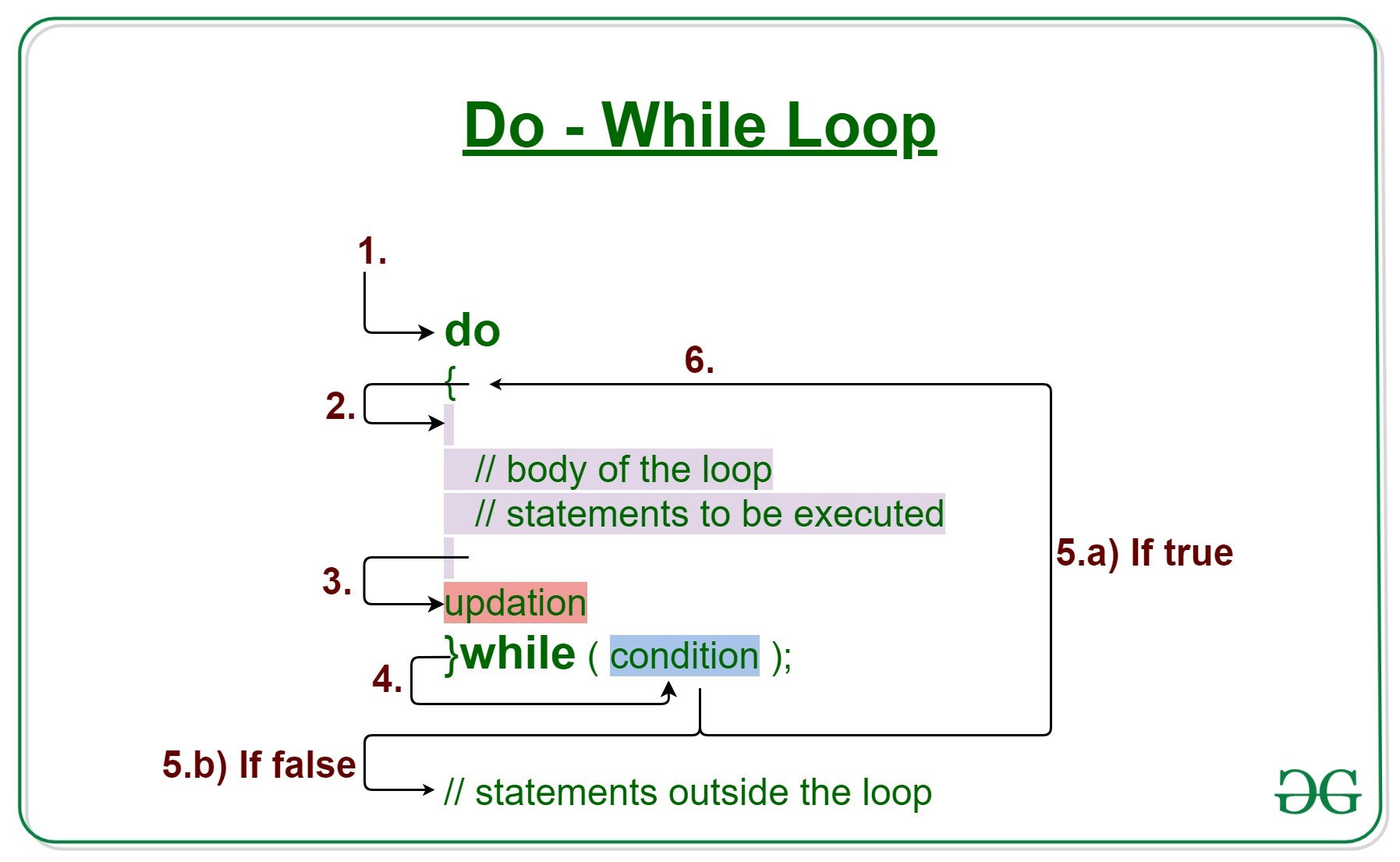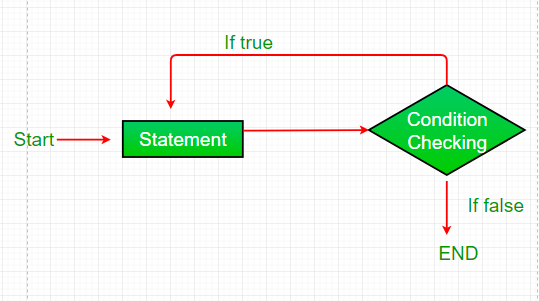GFG App
Open AppBrowser
Continue

# Java do-while loop with Examples

Loops in Java come into use when we need to repeatedly execute a block of statements. Java do-while loop is an Exit control loop. Therefore, unlike for or while loop, a do-while check for the condition after executing the statements of the loop body.

Syntax:

```do
{
// Loop Body
Update_expression
}

// Condition check
while (test_expression);```

Note: The test_expression for the do-while loop must return a boolean value , else we would get compile-time error.

Application of do-while : Its example application is showing some kind of menu to the users.

For example:

You are implementing a game where you show some options to the user, press 1 to do this .., press 2 to do this .. etc and press ‘Q’ to quit the game. So here you want to show the game menu to the user at least once, so you write the code for the game menu inside the do-while loop.Illustration:

## Java

 `// Java Program to Illustrate One Time Iteration` `// Inside do-while Loop` `// When Condition IS Not Satisfied`   `// Class` `class` `GFG {`   `    ``// Main driver method` `    ``public` `static` `void` `main(String[] args)` `    ``{` `        ``// initial counter variable` `        ``int` `i = ``0``;`   `        ``do` `{`   `            ``// Body of loop that will execute minimum` `            ``// 1 time for sure no matter what` `            ``System.out.println(``"Print statement"``);` `            ``i++;` `        ``}`   `        ``// Checking condition` `        ``// Note: It is being checked after` `        ``// minimum 1 iteration` `        ``while` `(i < ``0``);` `    ``}` `}`

Output

`Print statement`

Output explanation:

In the above code, we figured out that the condition is checked later as the body inside do will get executed one time without fail as the condition is checked later onwards. Hence whenever we want to display the menu and later on proceed command on the terminal, we always use do-while loop.

### Components of do-while Loop

A. Test Expression: In this expression, we have to test the condition. If the condition evaluates to true then we will execute the body of the loop and go to update expression. Otherwise, we will exit from the while loop. For example:

`i <= 10`

B. Update Expression: After executing the loop body, this expression increments/decrements the loop variable by some value. For example:

`i++;`

### Execution of do-While loop

1. Control falls into the do-while loop.
2. The statements inside the body of the loop get executed.
3. Updation takes place.
4. The flow jumps to Condition
5. Condition is tested.
1. If Condition yields true, go to Step 6.
2. If Condition yields false, the flow goes outside the loop
6. The flow goes back to Step 2.

### Flowchart do-while loop:Implementation:

Example 1: This program will try to print “Hello World” 5 times.

## Java

 `// Java Program to Illustrate Do-while Loop`   `// Class` `class` `GFG {`   `    ``// Main driver method` `    ``public` `static` `void` `main(String args[])` `    ``{`   `        ``// Declaring and initialization expression` `        ``int` `i = ``1``;`   `        ``// Do-while loop` `        ``do` `{`   `            ``// Body of do-while loop` `            ``// Print statement` `            ``System.out.println(``"Hello World"``);`   `            ``// Update expression` `            ``i++;` `        ``}`   `        ``// Test expression` `        ``while` `(i < ``6``);` `    ``}` `}`

Output:

```Hello World
Hello World
Hello World
Hello World
Hello World```

Auxiliary Space: O(1)

Output explanation:

The program will execute in the following manner as follows:

1. Program starts.
2. i is initialized with value 1.
3. Execution enters the loop
• “Hello World” gets printed 1st time.
• Updation is done. Now i = 2.
4. Condition is checked. 2 < 6 yields true.
5. Execution enters the loop.
• “Hello World” gets printed 2nd time.
• Updation is done. Now i = 3.
6. Condition is checked. 3 < 6 yields true.
7. Execution enters the loop
• “Hello World” gets printed 3rd time
• Updation is done. Now i = 4.
8. Condition is checked. 4 < 6 yields true.
9. Execution enters the loop
• “Hello World” gets printed 4th time
• Updation is done. Now i = 5.
10. Condition is checked. 5 < 6 yields true.
11. Execution enters the loop
• “Hello World” gets printed 5th time
• Updation is done. Now i = 6.
12. Condition is checked. 6 < 6 yields false.
13. The flow goes outside the loop.

Example 2

## Java

 `// Java Program to Illustrate Do-while Loop`   `// Class` `class` `GFG {`   `    ``// Main driver method` `    ``public` `static` `void` `main(String args[])` `    ``{` `        ``// Declaring and initializing integer values` `        ``int` `x = ``21``, sum = ``0``;`   `        ``// Do-while loop` `        ``do` `{`   `            ``// Execution statements(Body of loop)`   `            ``// Here, the line will be printed even` `            ``// if the condition is false` `            ``sum += x;` `            ``x--;` `        ``}`   `        ``// Now checking condition` `        ``while` `(x > ``10``);`   `        ``// Summing up` `        ``System.out.println(``"Summation: "` `+ sum);` `    ``}` `}`

Output:

`Summation: 176`

Example 3: do-while loop without curly braces {}

## Java

 `/*package whatever //do not write package name here */`   `import` `java.io.*;`   `class` `GFG {` `    ``public` `static` `void` `main (String[] args) {` `      ``int` `i=``1``;` `      ``do` `        ``// only single statement in do block` `        ``System.out.println(``"Hello GFG!"``);` `      ``// this condition is false so only do block will execute` `      ``while``(i>=``3``);` `      `  `      `  `    ``}` `}`

Output

```Hello GFG!
```

&list=PLqM7alHXFySF5ErEHA1BXgibGg7uqmA4_&ab_channel=GeeksforGeeks

Related Articles:

My Personal Notes arrow_drop_up